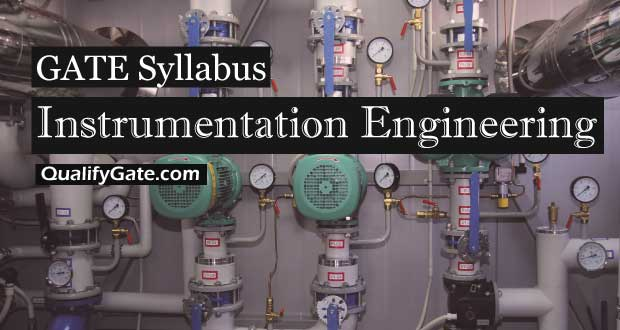# GATE 2018 Syllabus for Instrumentation Engineering (IN)

Last Updated: 16th March, 2017GATE 2018 Syllabus for Instrumentation Engineering pdf file download link is given below.

Syllabus for General Aptitude (GA) (common to all papers)

### Section 1: Engineering Mathematics

Linear Algebra: Matrix algebra, systems of linear equations, Eigen values and Eigen vectors.

Calculus: Mean value theorems, theorems of integral calculus, partial derivatives, maxima and minima, multiple integrals, Fourier series, vector identities, line, surface and volume integrals, Stokes, Gauss and Green’s theorems.

Differential equations: First order equation (linear and nonlinear), higher order linear differential equations with constant coefficients, method of variation of parameters, Cauchy’s and Euler’s equations, initial and boundary value problems, solution of partial differential equations: variable separable method.

Analysis of complex variables: Analytic functions, Cauchy’s integral theorem and integral formula, Taylor’s and Laurent’s series, residue theorem, solution of integrals.

Probability and Statistics: Sampling theorems, conditional probability, mean, median, mode and standard deviation, random variables, discrete and continuous distributions: normal, Poisson and binomial distributions.

Numerical Methods: Matrix inversion, solutions of non-linear algebraic equations, iterative methods for solving differential equations, numerical integration, regression and correlation analysis.

GATE 2018 Syllabus for Instrumentation Engineering Section 2

### Section 2: Electrical Circuits

Voltage and current sources: independent, dependent, ideal and practical; v-i relationships of resistor, inductor, mutual inductor and capacitor; transient analysis of RLC circuits with dc excitation.

Kirchoff’s laws, mesh and nodal analysis, superposition, Thevenin, Norton, maximum power transfer and reciprocity theorems.

Peak-, average- and rms values of ac quantities; apparent-, active- and reactive powers; phasor analysis, impedance and admittance; series and parallel resonance, locus diagrams, realization of basic filters with R, L and C elements.

One-port and two-port networks, driving point impedance and admittance, open-, and short circuit parameters.

GATE 2018 Syllabus for Instrumentation Engineering Section 3

### Section 3: Signals and Systems

Periodic, aperiodic and impulse signals; Laplace, Fourier and z-transforms; transfer function, frequency response of first and second order linear time invariant systems, impulse response of systems; convolution, correlation. Discrete time system: impulse response, frequency response, pulse transfer function; DFT and FFT; basics of IIR and FIR filters.

GATE 2018 Syllabus for Instrumentation Engineering Section 4

### Section 4: Control Systems

Feedback principles, signal flow graphs, transient response, steady-state-errors, Bode plot, phase and gain margins, Routh and Nyquist criteria, root loci, design of lead, lag and lead-lag compensators, state-space representation of systems; time-delay systems; mechanical, hydraulic and pneumatic system components, synchro pair, servo and stepper motors, servo valves; on-off, P, P-I, P-I-D, cascade, feedforward, and ratio controllers.

GATE 2018 Syllabus for Instrumentation Engineering Section 5

### Section 5: Analog Electronics

Characteristics and applications of diode, Zener diode, BJT and MOSFET; small signal analysis of transistor circuits, feedback amplifiers. Characteristics of operational amplifiers; applications of opamps: difference amplifier, adder, subtractor, integrator, differentiator, instrumentation amplifier, precision rectifier, active filters and other circuits. Oscillators, signal generators, voltage controlled oscillators and phase locked loop.

GATE 2018 Syllabus for Instrumentation Engineering Section 6

### Section 6: Digital Electronics

Combinational logic circuits, minimization of Boolean functions. IC families: TTL and CMOS. Arithmetic circuits, comparators, Schmitt trigger, multi-vibrators, sequential circuits, flip- flops, shift registers, timers and counters; sample-and-hold circuit, multiplexer, analog-to- digital (successive approximation, integrating, flash and sigma-delta) and digital-to- analog converters (weighted R, R-2R ladder and current steering logic). Characteristics of ADC and DAC (resolution, quantization, significant bits, conversion/settling time); basics of number systems, 8-bit microprocessor and microcontroller: applications, memory and input-output interfacing; basics of data acquisition systems.

GATE 2018 Syllabus for Instrumentation Engineering Section 7

### Section 7: Measurements

SI units, systematic and random errors in measurement, expression of uncertainty – accuracy and precision index, propagation of errors. PMMC, MI and dynamometer type instruments; dc potentiometer; bridges for measurement of R, L and C, Q-meter. Measurement of voltage, current and power in single and three phase circuits; ac and dc current probes; true rms meters, voltage and current scaling, instrument transformers, timer/counter, time, phase and frequency measurements, digital voltmeter, digital multimeter; oscilloscope, shielding and grounding.

GATE 2018 Syllabus for Instrumentation Engineering Section 8

### Section 8: Sensors and Industrial Instrumentation

Resistive, capacitive, inductive, piezoelectric, Hall effect sensors and associated signal conditioning circuits; transducers for industrial instrumentation: displacement (linear and angular), velocity, acceleration, force, torque, vibration, shock, pressure (including low pressure), flow (differential pressure, variable area, electromagnetic, ultrasonic, turbine and open channel flow meters) temperature (thermocouple, bolometer, RTD (3/4 wire), thermistor, pyrometer and semiconductor); liquid level, pH, conductivity and viscosity measurement.

GATE 2018 Syllabus for Instrumentation Engineering Section 9

### Section 9: Communication and Optical Instrumentation

Amplitude- and frequency modulation and demodulation; Shannon’s sampling theorem, pulse code modulation; frequency and time division multiplexing, amplitude-, phase-, frequency-, pulse shift keying for digital modulation; optical sources and detectors: LED, laser, photo-diode, light dependent resistor and their characteristics; interferometer: applications in metrology; basics of fiber optic sensing.